# farcar – Asemic

Just the other day I began to design a new asemic writing system using generative procedures. I hopped on over to my Computation for Creative Practice directory and began setting up project files in Processing to do so.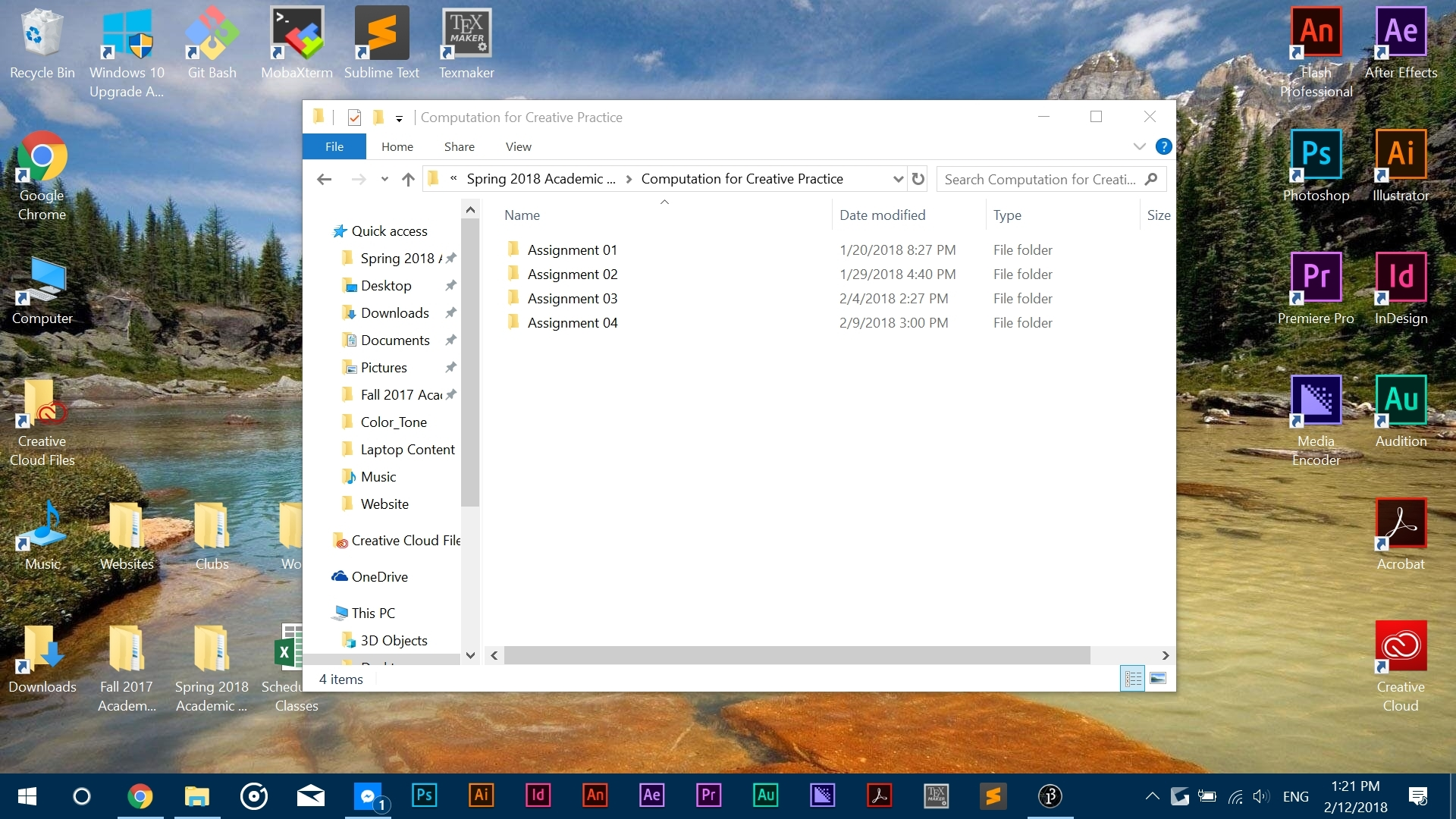Yet as I was programming an algorithm, I got an odd error message…The error message would not go away unless I clicked the Okay button, which I did. I had to get back to programming.

But soon enough, my computer started acting strange. It started glitching. I was able to take screenshots before it went away.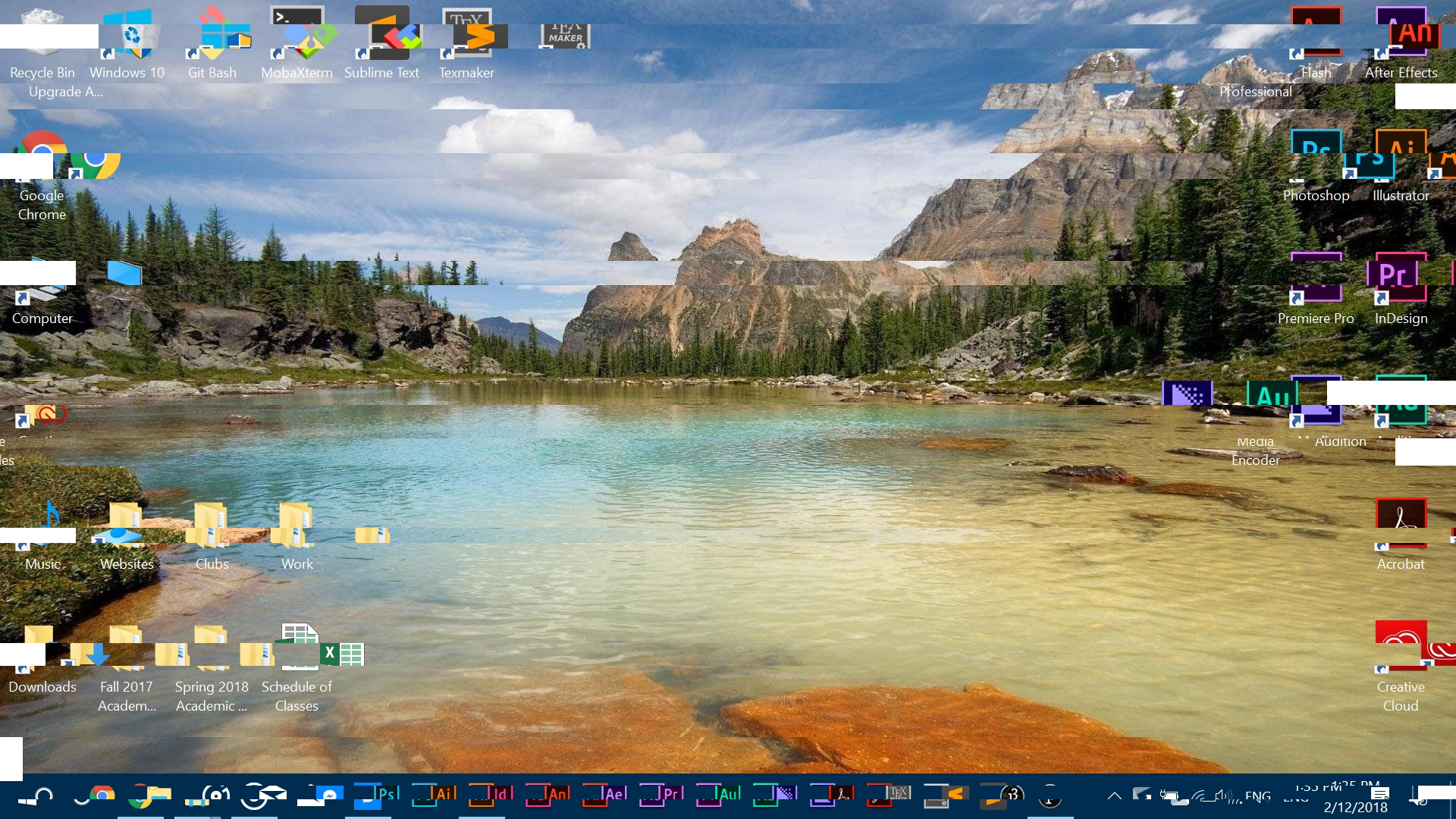And just like that, my computer went black.

But it wasn’t broken.

Soon enough, a new screen popped up. It was black, with this unreadable green text. I wanted to take a screenshot, but I didn’t have any screenshot tools with me.

These files, they must have been system files. As I went through them one by one, I realized they were the computer’s language. It was how the computer communicated with itself.

I grabbed my phone real quick to take a picture of the screen. I had to show someone.

Right as I took the image, my computer shut down. It then began to reboot. My computer had gone back to normal. But I still had the picture.

Using processing, I generated an algorithm that would reproduce the text in the image. After careful analysis, I realized that each character was just a binary representation of the English alphabet.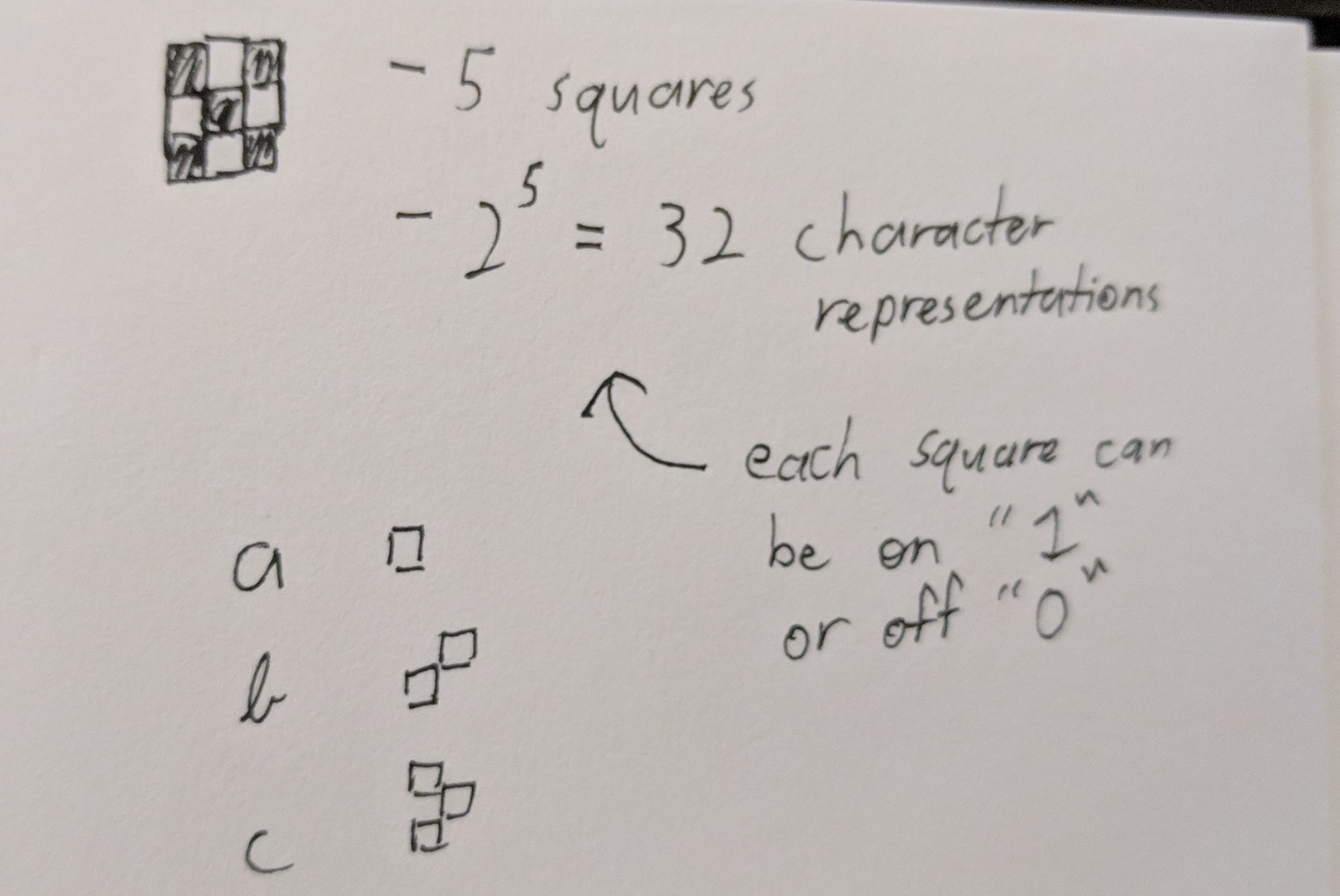Through this construction, a computer can easily read characters represented in binary that take up little space as opposed to reading the long string of 0’s and 1’s.

Below is the entire Processing sketch: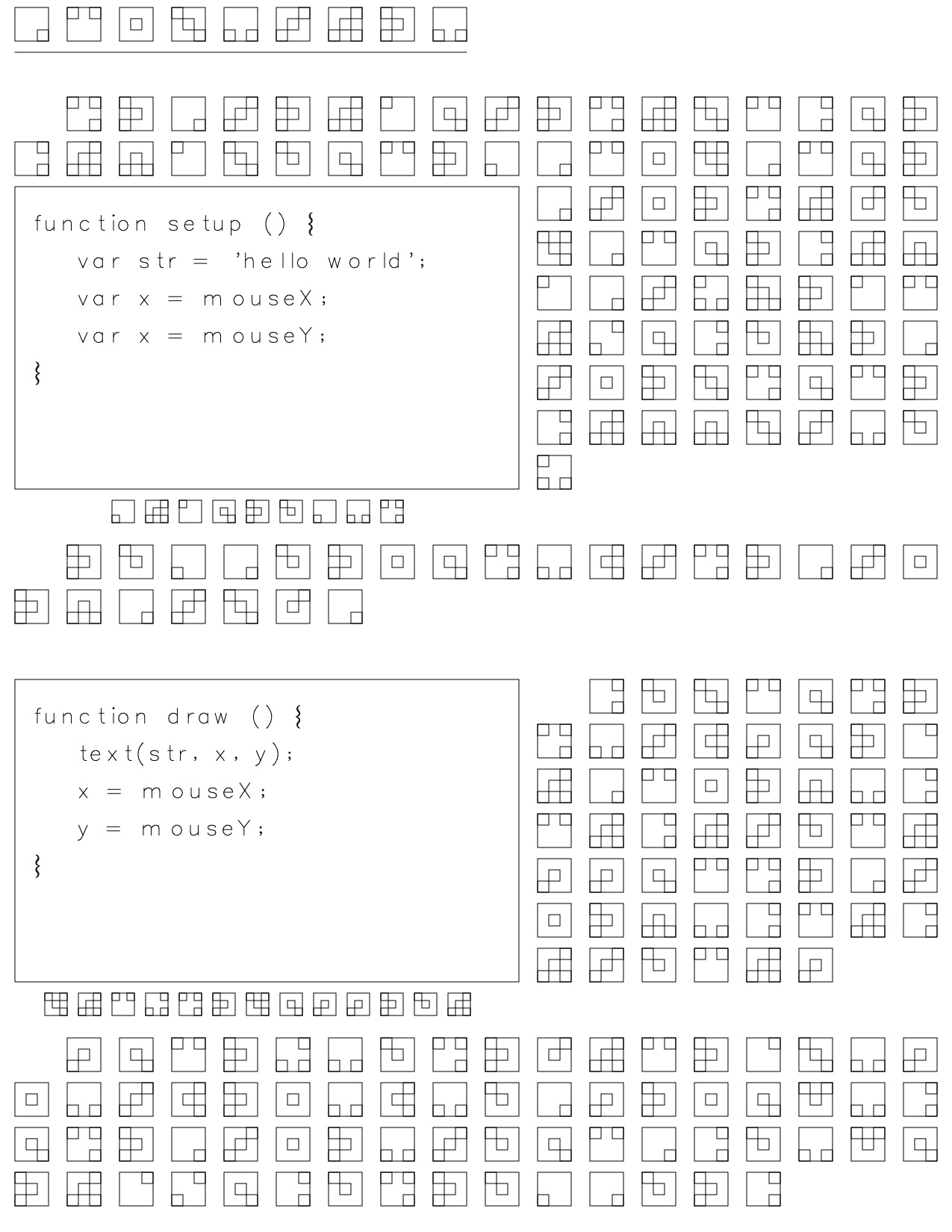Even computers mess up with their code too (see setup() line 3)
And after some help from Golan Levin & friends from the Studio, I was able to reproduce the image in print from a plotter.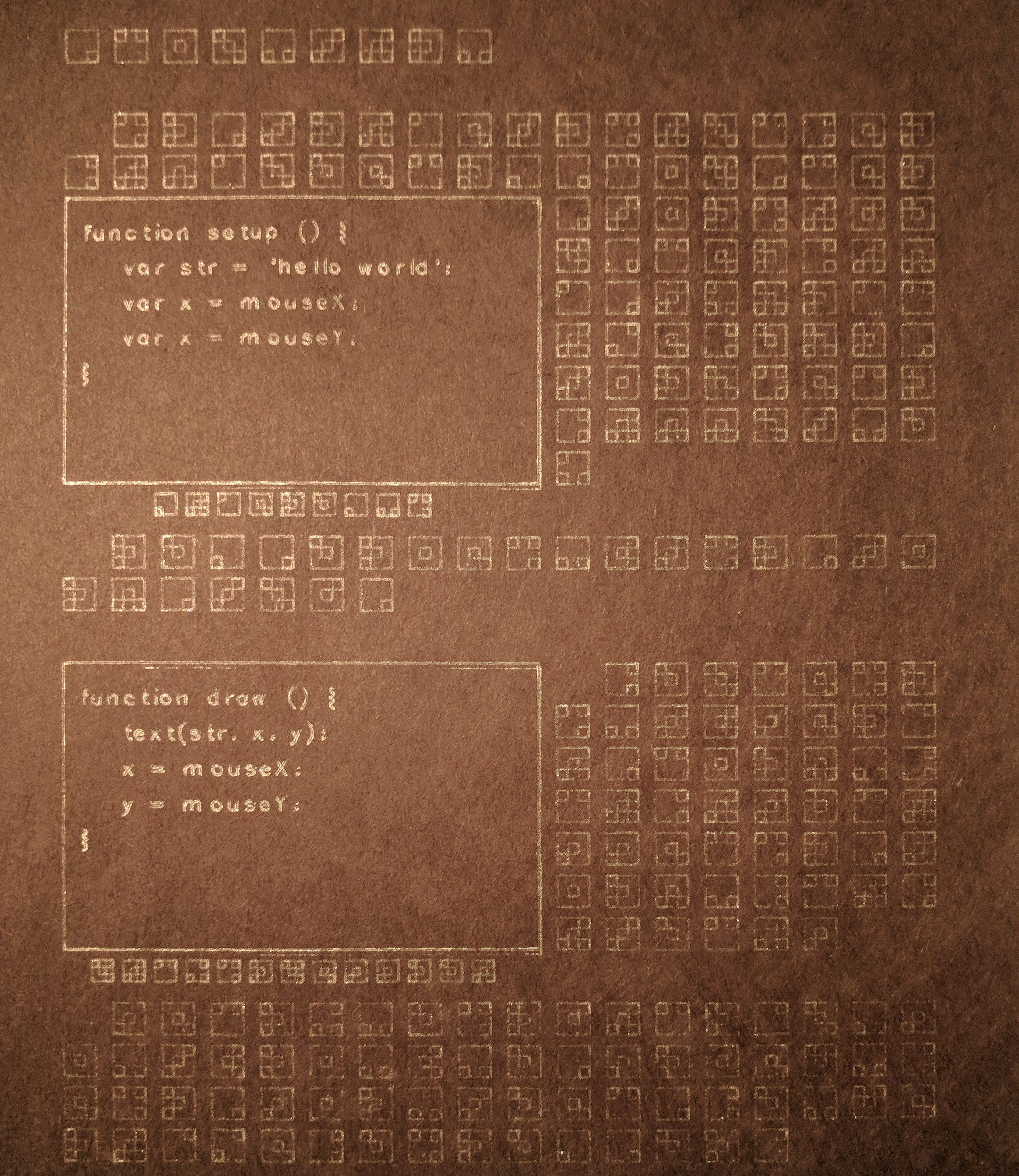The ink only shows well in light, giving way to the encryptic nature of the source image itself.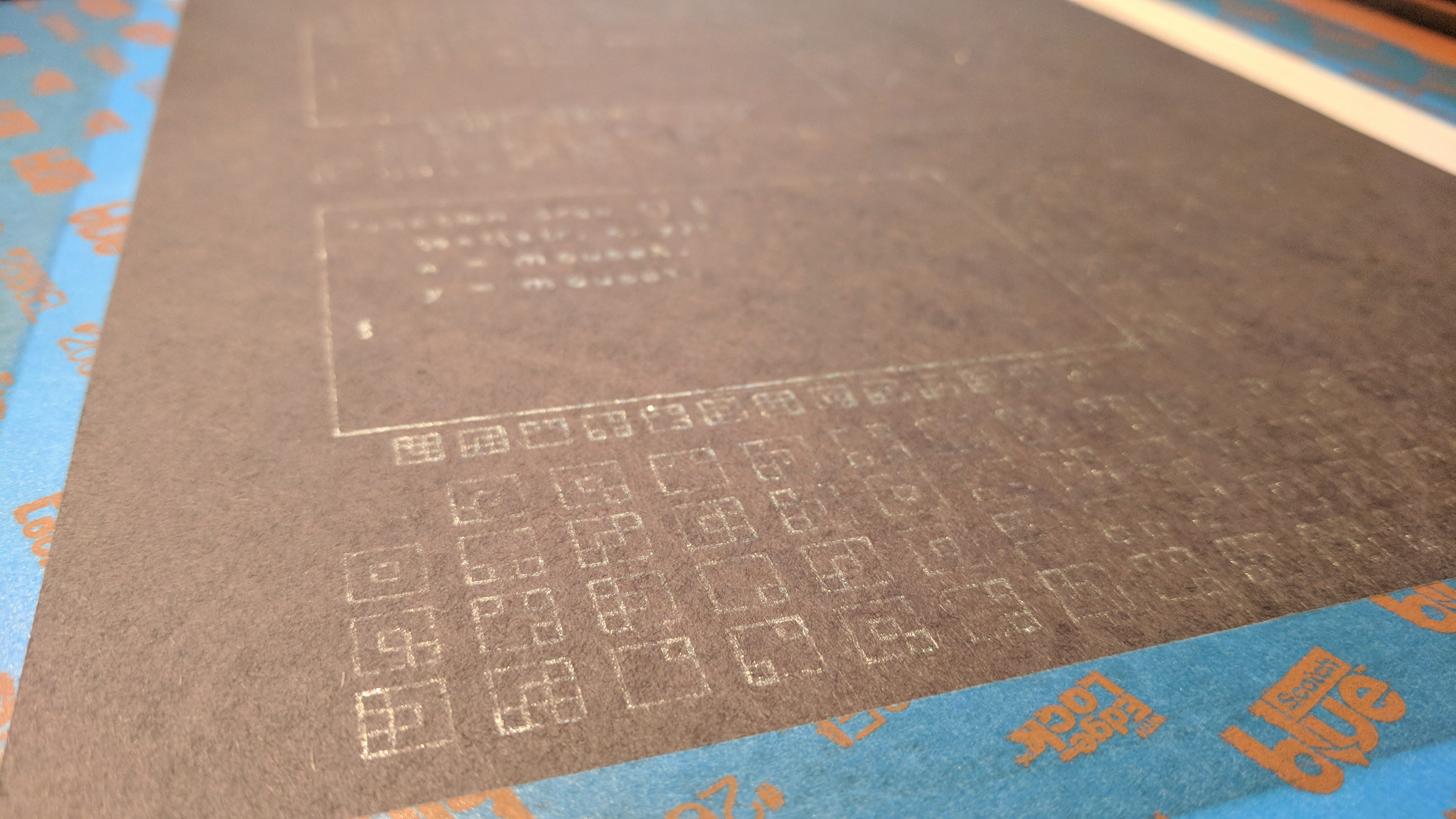Upon closer inspection, we can see that the image is the computer’s way of interpreting P5.js. Just like how we humans have manuals for understanding P5.js, so does the computer. It even has some helpful diagrams on the left side to help show what the code we give it looks like.In converting this image from the computer to print, I was much more mindful of what I was drawing and how I was drawing it: what I was seeing on the screen did not always guarantee the same result on print. One of the obstacles I had was representing English fonts. Currently, normal fonts in Processing are represented as fills, not outlines. To counter this, Golan was able to show me the Hershey one line fonts, to which I quickly found a working Processing implementation. Using the font, I was able to successful print the text.

Another conflict I found was with the physical materials I use. I am a digital artist, and as such, I do not have much experience with pens and brushes. It turns out, as you would never guess, pens and brushes don’t always work as do pixels do when drawing shapes you ask them to do. Below is an image I used with a thicker pen, where the ink would blob up due to the limited friction and high pressure of the plotter. I had to switch pens because of this to get better and finer results.Code

```//PDF saver template courtesy of Golan Levin //Hershey fonts from -> http://ixd-hof.de/processing_hersheyfont/ import processing.pdf.*; import de.ixdhof.hershey.*;   HersheyFont hf;   boolean bRecordingPDF; int pdfOutputCount; PVector[] imgBorders = new PVector; char[] character = {'a','b','c','d','e','f','g','h','i','j','k','l','m','n','o','p','q','r','s','t','u','v','w','x','y','z','.',' ',','}; String str = "Arduino is an open source computer hardware and software company, project, and user community that designs and manufactures single board microcontrollers and microcontroller kits for building digital devices and interactive objects that can sense and control objects in the physical world. Arduino is an open source computer hardware and software company, project, and user community that designs and manufactures single board microcontrollers and microcontroller kits for building digital devices and interactive objects that can sense and control objects in the physical world."; String newStr = str.toLowerCase(); char[] charsPressed = newStr.toCharArray(); int charsPressedIndx;   String sub01 = "hope this"; String newSub01 = sub01.toLowerCase(); char[] subChars01 = newSub01.toCharArray(); int subCharsIndx01;   String sub02 = "works well to"; String newSub02 = sub02.toLowerCase(); char[] subChars02 = newSub02.toCharArray(); int subCharsIndx02;   int[][] chars = { {0,0,0,0,1}, {0,0,0,1,0}, {0,0,0,1,1}, {0,0,1,0,0}, {0,0,1,0,1}, {0,0,1,1,0}, {0,0,1,1,1}, {0,1,0,0,0}, {0,1,0,0,1}, {0,1,0,1,0}, {0,1,0,1,1}, {0,1,1,0,0}, {0,1,1,0,1}, {0,1,1,1,0}, {0,1,1,1,1}, {1,0,0,0,0}, {1,0,0,0,1}, {1,0,0,1,0}, {1,0,0,1,1}, {1,0,1,0,0}, {1,0,1,0,1}, {1,0,1,1,0}, {1,0,1,1,1}, {1,1,0,0,0}, {1,1,0,0,1}, {1,1,0,1,0}, {1,1,0,1,1}, {1,1,1,0,0}, {1,1,1,0,1}, {1,1,1,1,0}, {1,1,1,1,1}, };   void setup() { size(1275,1650);   hf = new HersheyFont(this, "futural.jhf"); hf.textSize(20);   imgBorders = new PVector(20, 250); imgBorders = new PVector(20, 910); charsPressedIndx = charsPressed.length; subCharsIndx01 = subChars01.length; subCharsIndx02 = subChars02.length; bRecordingPDF = false; pdfOutputCount = 0; noFill(); stroke(1); }   void draw() { background(255); if(bRecordingPDF) beginRecord(PDF, "myName_" + pdfOutputCount + ".pdf");   noFill(); for(int i = 0; i < imgBorders.length; i++) rect(imgBorders[i].x, imgBorders[i].y, 675, 405); textSize(25); hf.text("function setup () {", 50, 300); hf.text(" var str = 'hello world';", 50, 350); hf.text(" var x = mouseX;", 50, 400); hf.text(" var x = mouseY;", 50, 450); hf.text("}", 50, 500); hf.text("function draw () {", 50, 960); hf.text(" text(str, x, y);", 50, 1010); hf.text(" x = mouseX;", 50, 1060); hf.text(" y = mouseY;", 50, 1110); hf.text("}", 50, 1160); small_keys01(); small_keys02(); draw_keys(); line(20, 70, 625, 70);   if(bRecordingPDF) { endRecord(); bRecordingPDF = false; pdfOutputCount++; } }   void keyPressed() { int i = keyType(key); if(i != -1) { charsPressed[charsPressedIndx] = key; charsPressedIndx++; } else if(key == BACKSPACE) if(charsPressedIndx > 0) charsPressedIndx--; }   int keyType(char chr) { for(int i = 0; i < character.length; i++) { if(character[i] == chr) return i; } return -1; }   void small_keys01() { int x_indx = 150; int y_indx = 670; for(int j = 0; j < subCharsIndx01; j++) { int i = keyType(subChars01[j]); if(chars[i] == 1) rect(x_indx, y_indx, 10, 10); if(chars[i] == 1) rect(x_indx, y_indx+20, 10, 10); if(chars[i] == 1) rect(x_indx+10, y_indx+10, 10, 10); if(chars[i] == 1) rect(x_indx+20, y_indx, 10, 10); if(chars[i] == 1) rect(x_indx+20, y_indx+20, 10, 10); rect(x_indx, y_indx, 30, 30); x_indx += 45; } }   void small_keys02() { int x_indx = 60; int y_indx = 1330; for(int j = 0; j < subCharsIndx02; j++) { int i = keyType(subChars02[j]); if(chars[i] == 1) rect(x_indx, y_indx, 10, 10); if(chars[i] == 1) rect(x_indx, y_indx+20, 10, 10); if(chars[i] == 1) rect(x_indx+10, y_indx+10, 10, 10); if(chars[i] == 1) rect(x_indx+20, y_indx, 10, 10); if(chars[i] == 1) rect(x_indx+20, y_indx+20, 10, 10); rect(x_indx, y_indx, 30, 30); x_indx += 45; } }   void draw_keys() { int x_indx = 20; int y_indx = 10; for(int j = 0; j < charsPressedIndx; j++) { int i = keyType(charsPressed[j]); if(chars[i] == 1) rect(x_indx, y_indx, 15, 15); if(chars[i] == 1) rect(x_indx, y_indx+30, 15, 15); if(chars[i] == 1) rect(x_indx+15, y_indx+15, 15, 15); if(chars[i] == 1) rect(x_indx+30, y_indx, 15, 15); if(chars[i] == 1) rect(x_indx+30, y_indx+30, 15, 15); rect(x_indx, y_indx, 45, 45); x_indx += 70; if(x_indx > 0.5*width && y_indx == 10) { x_indx = 90; y_indx += 120; } else if(x_indx > 0.6*width && y_indx == 610) { x_indx = 90; y_indx += 120; } else if(x_indx > 0.35*width && y_indx == 790) { x_indx = 790; y_indx += 120; } else if(x_indx > 0.85*width && y_indx == 1270) { x_indx = 90; y_indx += 120; } else if(y_indx + 120 > height && x_indx + 20 > 0.8*width) { y_indx += 120; } else if(x_indx + 20 > width) { x_indx = 20; y_indx += 60; } for(int k = 0; k < imgBorders.length; k++) while(x_indx+45 > imgBorders[k].x && x_indx < imgBorders[k].x+675 && y_indx+45 > imgBorders[k].y && y_indx < imgBorders[k].y+390) x_indx += 70; } }   void mousePressed() { bRecordingPDF = true; }```